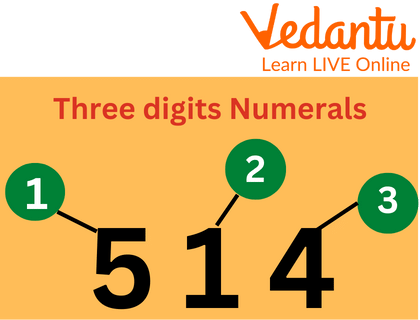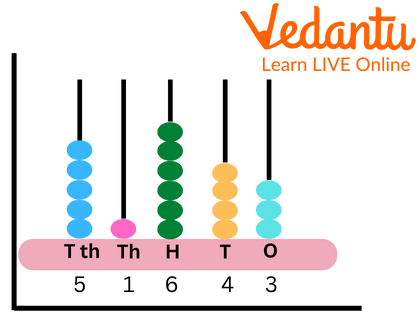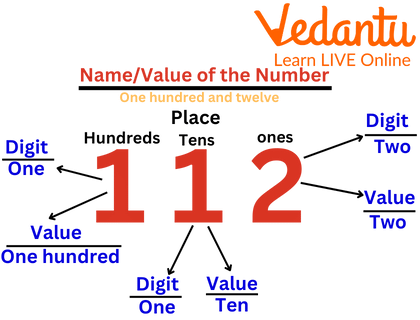Courses
Courses for Kids
Free study material
Offline Centres
More

# NumerationLast updated date: 08th Dec 2023
Total views: 114.6k
Views today: 1.14k## Numeration - Introduction

We use a set of digits known as numerals to represent numbers. A few examples of numbers are 1234 2314 56111. Numeration is the process of expressing numbers in words. is described as a logical writing system that uses digits or symbols to represent numbers. In this article, we will discuss numbers and numeration in detail.

## Number and Numeration

An arithmetic value used to indicate an amount is called a number. A number is a mathematical concept that is used for counting, measuring and labelling. Thus, Mathematics is built on numbers. Numeration is the process of expressing numbers in words. A number system is described as a logical writing system that uses digits or symbols to represent numbers.Examples of Numerals

## What is Numeration?

Numbers are represented by symbols or groupings of symbols called numerals. Expressing a number in words is called numeration. Numeration systems are organised ways or processes for counting to figure out how many total units are in a collection.

Three functions of numerical systems are identification, ordering and counting. When determining who crosses the finish line first, second or third in a race, for instance, numerical systems can also be used to identify a person's or unit's position in a series.Numeration with Abacus

## Difference between Number and Numeral

A number is a measurement or count that, in our minds, is essentially an idea. Numbers are expressed using numerals like "4" or "four" in writing or conversation. However, we might also touch the ground four times or hold up four fingers. These all serve as alternative expressions for the same number.

A numeral is a name or symbol that represents a certain number. Examples are the numerals 3, 49 and twelve. Therefore, the numeral is how we write the number.Value of a Number

## Solved Examples

Example 1: Which number will be produced by adding one to :

(i) 9

(ii) 99

Ans: (i) We will get 10 if 1 is added to 9.

(ii) If 1 is added to 99, we will get 100.

Example 2: Write the following in descending order:

(i) 308, 312, 306, 318

(ii) 513, 515, 510, 525

Ans: As we know that information is considered to be in descending order if it is arranged from highest to lowest.

(i) 318, 312, 308, 306

(ii) 525, 515, 513, 510

Example 3: Write the numbers that are both bigger and less than the specified number:

(i) More than 539 but less than 544

(ii) Less than 999 but more than 994

Ans: (i) A number which is more than 539 and less than 544 is 541.

(ii) A number which is less than 999 and more than 994 is 996.

## Conclusion

The technique of representing a number in digits or figures is known as notation. Numeration is the process of representing numbers in words. We have learned about numbers and numeration in detail in this article. We have also provided some solved examples to understand the topics better.

## FAQs on Numeration

1. What are numbers?

A number is an arithmetic value that is used to calculate and represent a quantity. A number system is a logical way of writing numbers that uses digits or symbols to represent them.

2. Which is the smallest one-digit number?

The smallest one-digit number is 0 (zero).

3. What is the largest two-digit number?

The largest two-digit number is 99.